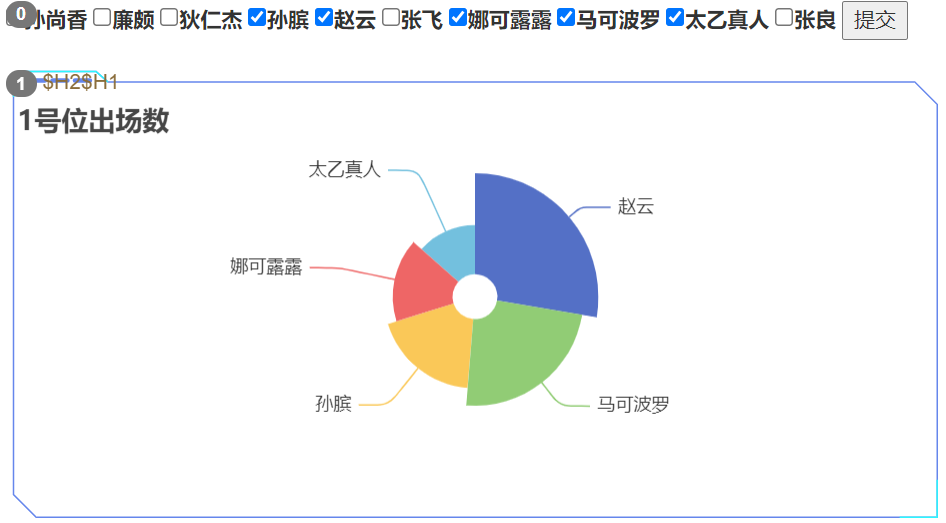# SMARTCHART多项筛选联动

Smartchart对于单项筛选有通用的组件， 可以直接配置即可，但对于个性化的筛选，需要你进行一些简单的定制化，以下就针对多项筛选联动的需求来介绍如何定制化你的筛选联动效果以我们内置的仪表盘为例，

```select distinct H1 as heroname  from smartdemo2 limit 10
```

```//select distinct xx  from tablename
let dataset=__dataset__;
let table = '';
for (let i=1;i<dataset.length;i++){
table = `\${table}<label><input name="select__name__" type="checkbox" value="\${dataset[i]}" />\${dataset[i]}</label> `;
}
table = table + "<button id='id_select__name__'>提交</button>";

dom__name__.innerHTML=table;

\$('#id_select__name__').click(
function(){
let res = [];
\$("input[name='select__name__']:checked").each(function(i){
res.push("'" + \$(this).val() + "'");
});

filter_param['H1'] = res.toString();  //H1是参数名
ds_refresh(1); //刷新图形1

}
)
```

```select H1 as heroname, sum(qty) as 出场数 from smartdemo2
where 1=1
/* and H2 = '\$H2' */
/* and H1 in (\$H1) */      --此处来新增
group by H1
order by sum(qty) desc
```